## Overview: LP Solver

The OPTMODEL procedure provides a framework for specifying and solving linear programs (LPs). A standard linear program has the following formulation: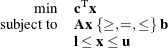where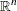is the vector of decision variables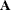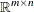is the matrix of constraintsis the vector of objective function coefficients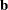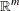is the vector of constraints right-hand sides (RHS)is the vector of lower bounds on variablesis the vector of upper bounds on variables

The following LP solvers are available in the OPTMODEL procedure:

• primal simplex solver

• dual simplex solver

• network simplex solver

• interior point solver

The primal and dual simplex solvers implement the two-phase simplex method. In phase I, the solver tries to find a feasible solution. If no feasible solution is found, the LP is infeasible; otherwise, the solver enters phase II to solve the original LP. The network simplex solver extracts a network substructure, solves this using network simplex, and then constructs an advanced basis to feed to either primal or dual simplex. The interior point solver implements a primal-dual predictor-corrector interior point algorithm. If any of the decision variables are constrained to be integer-valued, then the relaxed version of the problem is solved.